Skip to content →

# Tag: puzzle

The buckyball is without doubt the hottest mahematical object at the moment (at least in Europe). Recall that the buckyball (middle) is a mixed form of two Platonic solids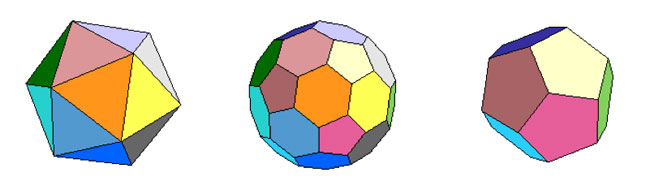the Icosahedron on the left and the Dodecahedron on the right.

For those of you who don’t know anything about football, it is that other ball-game, best described via a quote from the English player Gary Lineker

“Football is a game for 22 people that run around, play the ball, and one referee who makes a slew of mistakes, and in the end Germany always wins.”

We still have a few days left hoping for a better ending… Let’s do some bucky-maths : what is the rotation symmetry group of the buckyball?

For starters, dodeca- and icosahedron are dual solids, meaning that if you take the center of every face of a dodecahedron and connect these points by edges when the corresponding faces share an edge, you’ll end up with the icosahedron (and conversely). Therefore, both solids (as well as their mixture, the buckyball) will have the same group of rotational symmetries. Can we at least determine the number of these symmetries?

Take the dodecahedron and fix a face. It is easy to find a rotation taking this face to anyone of its five adjacent faces. In group-slang : the rotation automorphism group acts transitively on the 12 faces of the dodecohedron. Now, how many of them fix a given face? These can only be rotations with axis through the center of the face and there are exactly 5 of them preserving the pentagonal face. So, in all we have $12 \times 5 = 60$ rotations preserving any of the three solids above. By composing two of its elements, we get another rotational symmetry, so they form a group and we would like to determine what that group is.

There is one group that springs to mind $A_5$, the subgroup of all even permutations on 5 elements. In general, the alternating group has half as many elements as the full permutation group $S_n$, that is $\frac{1}{2} n!$ (for multiplying with the involution (1,2) gives a bijection between even and odd permutations). So, for $A_5$ we get 60 elements and we can list them :

• the trivial permutation$~()$, being the identity.
• permutations of order two with cycle-decompostion $~(i_1,i_2)(i_3,i_4)$, and there are exactly 15 of them around when all numbers are between 1 and 5.
• permutations of order three with cycle-form $~(i_1,i_2,i_3)$ of which there are exactly 20.
• permutations of order 5 which have to form one full cycle $~(i_1,i_2,i_3,i_4,i_5)$. There are 24 of those.

Can we at least view these sets of elements as rotations of the buckyball? Well, a dodecahedron has 12 pentagobal faces. So there are 4 nontrivial rotations of order 5 for every 2 opposite faces and hence the dodecaheder (and therefore also the buckyball) has indeed 6×4=24 order 5 rotational symmetries.

The icosahedron has twenty triangles as faces, so any of the 10 pairs of opposite faces is responsible for two non-trivial rotations of order three, giving us 10×2=20 order 3 rotational symmetries of the buckyball.

The order two elements are slightly harder to see. The icosahedron has 30 edges and there is a plane going through each of the 15 pairs of opposite edges splitting the icosahedron in two. Hence rotating to interchange these two edges gives one rotational symmetry of order 2 for each of the 15 pairs.

And as 24+20+15+1(identity) = 60 we have found all the rotational symmetries and we see that they pair up nicely with the elements of $A_5$. But do they form isomorphic groups? In other words, can the buckyball see the 5 in the group $A_5$.

In a previous post I’ve shown that one way to see this 5 is as the number of inscribed cubes in the dodecahedron. But, there is another way to see the five based on the order 2 elements described before.

If you look at pairs of opposite edges of the icosahedron you will find that they really come in triples such that the planes determined by each pair are mutually orthogonal (it is best to feel this on ac actual icosahedron). Hence there are 15/3 = 5 such triples of mutually orthogonal symmetry planes of the icosahedron and of course any rotation permutes these triples. It takes a bit of more work to really check that this action is indeed the natural permutation action of $A_5$ on 5 elements.

Having convinced ourselves that the group of rotations of the buckyball is indeed the alternating group $A_5$, we can reverse the problem : can the alternating group $A_5$ see the buckyball???

Well, for starters, it can ‘see’ the icosahedron in a truly amazing way. Look at the conjugacy classes of $A_5$. We all know that in the full symmetric group $S_n$ elements belong to the same conjugacy class if and only if they have the same cycle decomposition and this is proved using the fact that the conjugation f a cycle $~(i_1,i_2,\ldots,i_k)$ under a permutation $\sigma \in S_n$ is equal to the cycle $~(\sigma(i_1),\sigma(i_2),\ldots,\sigma(i_k))$ (and this gives us also the candidate needed to conjugate two partitions into each other).

Using this trick it is easy to see that all the 15 order 2 elements of $A_5$ form one conjugacy class, as do the 20 order 3 elements. However, the 24 order 5 elements split up in two conjugacy classes of 12 elements as the permutation needed to conjugate $~(1,2,3,4,5)$ to $~(1,2,3,5,4)$ is $~(4,5)$ but this is not an element of $A_5$.

Okay, now take one of these two conjugacy classes of order 5 elements, say that of $~(1,2,3,4,5)$. It consists of 12 elements, 12 being also the number of vertices of the icosahedron. So, is there a way to identify the elements in the conjugacy class to the vertices in such a way that we can describe the edges also in terms of group-computations in $A_5$?

Surprisingly, this is indeed the case as is demonstrated in a marvelous paper by Kostant “The graph of the truncated icosahedron and the last letter of Galois”.

Two elements $a,b$ in the conjugacy class C share an edge if and only if their product $a.b \in A_5$ still belongs to the conjugacy class C!

So, for example $~(1,2,3,4,5).(2,1,4,3,5) = (2,5,4)$ so there is no edge between these elements, but on the other hand $~(1,2,3,4,5).(5,3,4,1,2)=(1,5,2,4,3)$ so there is an edge between these! It is no coincidence that $~(5,3,4,1,2)=(2,1,4,3,5)^{-1}$ as inverse elements correspond in the bijection to opposite vertices and for any pair of non-opposite vertices of an icosahedron it is true that either they are neighbors or any one of them is the neighbor of the opposite vertex of the other element.

If we take $u=(1,2,3,4,5)$ and $v=(5,3,4,1,2)$ (or any two elements of the conjugacy class such that u.v is again in the conjugacy class), then one can describe all the vertices of the icosahedron group-theoretically as follows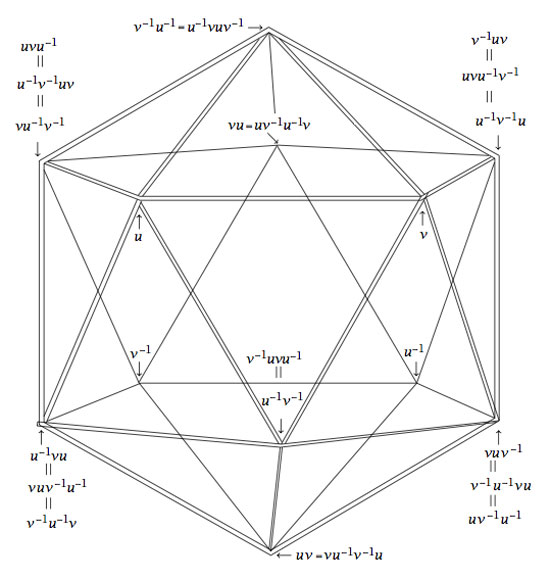Isn’t that nice? Well yes, you may say, but that is just the icosahedron. Can the group $A_5$ also see the buckyball?

Well, let’s try a similar strategy : the buckyball has 60 vertices, exactly as many as there are elements in the group $A_5$. Is there a way to connect certain elements in a group according to fixed rules? Yes, there is such a way and it is called the Cayley Graph of a group. It goes like this : take a set of generators ${ g_1,\ldots,g_k }$ of a group G, then connect two group element $a,b \in G$ with an edge if and only if $a = g_i.b$ or $b = g_i.a$ for some of the generators.

Back to the alternating group $A_5$. There are several sets of generators, one of them being the elements ${ (1,2,3,4,5),(2,3)(4,5) }$. In the paper mentioned before, Kostant gives an impressive group-theoretic proof of the fact that the Cayley-graph of $A_5$ with respect to these two generators is indeed the buckyball!

Let us allow to be lazy for once and let SAGE do the hard work for us, and let us just watch the outcome. Here’s how that’s done

A=PermutationGroup([‘(1,2,3,4,5)’,'(2,3)(4,5)’])
B=A.cayley_graph()
B.show3d()

The outcone is a nice 3-dimensional picture of the buckyball. Below you can see a still, and, if you click on it you will get a 3-dimensional model of it (first click the ‘here’ link in the new window and then you’d better control-click and set the zoom to 200% before you rotate it)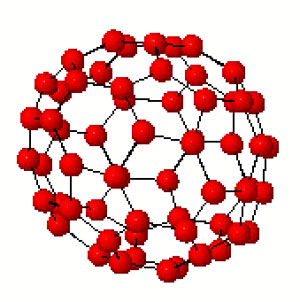Hence, viewing this Cayley graph from different points we have convinced ourselves that it is indeed the buckyball. In fact, most (truncated) Platonic solids appear as Cayley graphs of groups with respect to specific sets of generators. For later use here is a (partial) survey (taken from Jaap’s puzzle page)Tetrahedron : $C_2 \times C_2,[(12)(34),(13)(24),(14)(23)]$
Cube : $D_4,[(1234),(13)]$
Octahedron : $S_3,[(123),(12),(23)]$
Dodecahedron : IMPOSSIBLE
Icosahedron : $A_4,[(123),(234),(13)(24)]$Truncated tetrahedron : $A_4,[(123),(12)(34)]$
Cuboctahedron : $A_4,[(123),(234)]$
Truncated cube : $S_4,[(123),(34)]$
Truncated octahedron : $S_4,[(1234),(12)]$
Rhombicubotahedron : $S_4,[(1234),(123)]$
Rhombitruncated cuboctahedron : IMPOSSIBLE
Snub cuboctahedron : $S_4,[(1234),(123),(34)]$Icosidodecahedron : IMPOSSIBLE
Truncated dodecahedron : $A_5,[(124),(23)(45)]$
Truncated icosahedron : $A_5,[(12345),(23)(45)]$
Rhombicosidodecahedron : $A_5,[(12345),(124)]$
Rhombitruncated icosidodecahedron : IMPOSSIBLE
Snub Icosidodecahedron : $A_5,[(12345),(124),(23)(45)]$

Again, all these statements can be easily verified using SAGE via the method described before. Next time we will go further into the Kostant’s group-theoretic proof that the buckyball is the Cayley graph of $A_5$ with respect to (2,5)-generators as this calculation will be crucial in the description of the buckyball curve, the genus 70 Riemann surface discovered by David Singerman and
Pablo Martin which completes the trinity corresponding to the Galois trinityEver tried a chess problem like : White to move, mate in two! Of course you have, and these are pretty easy to solve : you only have to work through the finite list of white first moves and decide whether or not black has a move left preventing mate on the next white move. This is even a (non-optimal) fool-proof algorithm to find the solution to this kind of chess problems. Right?

Wrong! There exist concrete positions, provable mate in two in which it is NOT possible to determine the winning first move for white! So, what’s wrong with the argument above? We did assume that, given the position, it is possible to determine all legal moves for the two players. So?

Well, some moves are legal only depending on the history of the game. For example, you are only allowed to do a castling if your king nor your rook made a prior move. Further, you can only make an en-passant-capture on the next move.

But surely all this is just theoretical? No-one ever constructed a provable 2-mate with impossible winning move. Wrong again. The logician Raymond Smullyan did precisely that in his retro-chess puzzle book Chess mysteries of Sherlock Holmes. Here’s the position :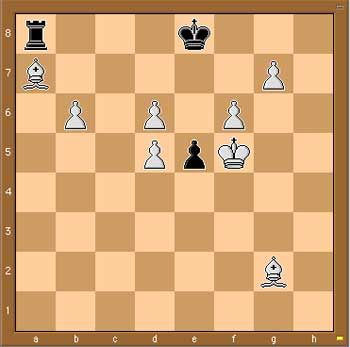Presumably every chess player goes for the mate : 1. Kf5-e6 2. g7-g8D But what if black counters your first move with a castling 1. … 0-0-0 ? Surely he isnt allowed to do this. Why not, is there any clue in the position to prove that either the king or the rook must have moved before?

Well, what was black previous move then? It cannot be the pawn move e6-e5 as before that move the white King would be in check, so what was it? Just one possibility left : it must have been e7-e5.

This offers then another winning strategy for white, as white can capture en-passant. 1. d5xe6 e.p. and then if black castles 1. … 0.0.0, 2. b6-b7 or is black does any other move : 2. g7-g8.

Hence, whatever the games’ history, white has a mate in two! However, looking ONLY at the given position, it is impossible for him to judge whether Kf5-e6 will do the trick!

Anyone seen similar constructions?

UPDATE

According to the wikipedia page on Retrograde chess analysis, the Smullyan-idea is an adaptation of a much older problem due to W. Langstaff in the Chess Amateur of 1922. Here’s the situation (the solution is the same as above)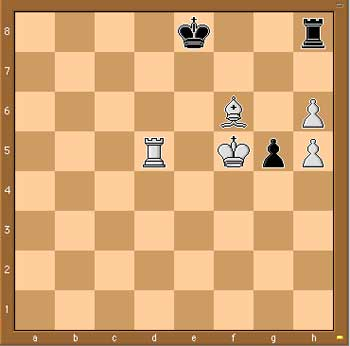MUBs (for Mutually Unbiased Bases) are quite popular at the moment. Kea is running a mini-series Mutual Unbias as is Carl Brannen. Further, the Perimeter Institute has a good website for its seminars where they offer streaming video (I like their MacromediaFlash format giving video and slides/blackboard shots simultaneously, in distinct windows) including a talk on MUBs (as well as an old talk by Wootters).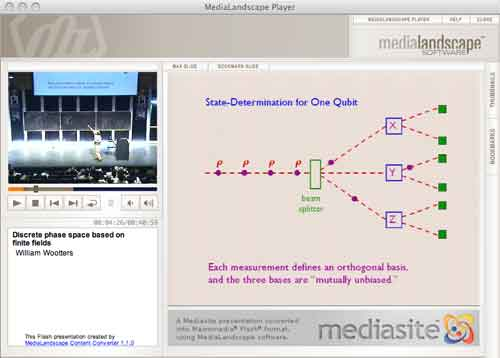So what are MUBs to mathematicians? Recall that a d-state quantum system is just the vectorspace $\mathbb{C}^d$ equipped with the usual Hermitian inproduct $\vec{v}.\vec{w} = \sum \overline{v_i} w_i$. An observable $E$ is a choice of orthonormal basis ${ \vec{e_i} }$ consisting of eigenvectors of the self-adjoint matrix $E$. $E$ together with another observable $F$ (with orthonormal basis ${ \vec{f_j} }$) are said to be mutally unbiased if the norms of all inproducts $\vec{f_j}.\vec{e_i}$ are equal to $1/\sqrt{d}$. This definition extends to a collection of pairwise mutually unbiased observables. In a d-state quantum system there can be at most d+1 mutually unbiased bases and such a collection of observables is then called a MUB of the system. Using properties of finite fields one has shown that MUBs exists whenever d is a prime-power. On the other hand, existence of a MUB for d=6 still seems to be open…

The King’s Problem (( actually a misnomer, it’s more the poor physicists’ problem… )) is the following : A physicist is trapped on an island ruled by a mean
king who promises to set her free if she can give him the answer to the following puzzle. The
physicist is asked to prepare a d−state quantum system in any state of her choosing and give it
to the king, who measures one of several mutually unbiased observables on it. Following this, the physicist is allowed to make a control measurement
on the system, as well as any other systems it may have been coupled to in the preparation
phase. The king then reveals which observable he measured and the physicist is required
to predict correctly all the eigenvalues he found.

The Solution to the King’s problem in prime power dimension by P. K. Aravind, say for $d=p^k$, consists in taking a system of k object qupits (when $p=2l+1$ one qupit is a spin l particle) which she will give to the King together with k ancilla qupits that she retains in her possession. These 2k qupits are diligently entangled and prepared is a well chosen state. The final step in finding a suitable state is the solution to a pure combinatorial problem :

She must use the numbers 1 to d to form $d^2$ ordered sets of d+1 numbers each, with repetitions of numbers within a set allowed, such that any two sets have exactly one identical number in the same place in both. Here’s an example of 16 such strings for d=4 :

11432, 12341, 13214, 14123, 21324, 22413, 23142, 24231, 31243, 32134, 33421, 34312, 41111, 42222, 43333, 44444

Here again, finite fields are used in the solution. When $d=p^k$, identify the elements of $\mathbb{F}_{p^k}$ with the numbers from 1 to d in some fixed way. Then, the $d^2$ of number-strings are found as follows : let $k_0,k_1 \in \mathbb{F}_{p^k}$ and take as the first 2 numbers the ones corresponding to these field-elements. The remaning d-2 numbers in the string are those corresponding to the field element $k_m$ (with $2 \leq m \leq d$) determined from $k_0,k_1$ by the equation

$k_m = l_{m} * k_0+k_1$

where $l_i$ is the field-element corresponding to the integer i ($l_1$ corresponds to the zero element). It is easy to see that these $d^2$ strings satisfy the conditions of the combinatorial problem. Indeed, any two of its digits determine $k_0,k_1$ (and hence the whole string) as it follows from
$k_m = l_m k_0 + k_1$ and $k_r = l_r k_0 + k_1$ that $k_0 = \frac{k_m-k_r}{l_m-l_r}$.

In the special case when d=3 (that is, one spin 1 particle is given to the King), we recover the tetracode : the nine codewords

0000, 0+++, 0—, +0+-, ++-0, +-0+, -0-+, -+0-, –+0

encode the strings (with +=1,-=2,0=3)

3333, 3111, 3222, 1312, 1123, 1231, 2321, 2132, 2213

The Archimedes codex is a good read, especially when you are (like me) a failed archeologist. The palimpsest (Greek for ‘scraped again’) is the worlds first Kyoto-approved ‘sustainable writing’. Isn’t it great to realize that one of the few surviving texts by Archimedes only made it because some monks recycled an old medieval parchment by scraping off most of the text, cutting the pages in half, rebinding them and writing a song-book on them…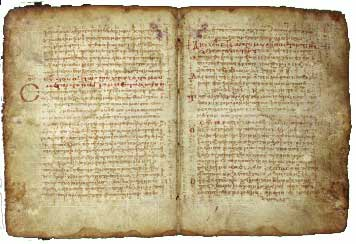The Archimedes-text is barely visible as vertical lines running through the song-lyrics. There is a great website telling the story in all its detail.

Contrary to what the books claims I don’t think we will have to rewrite maths history. Didn’t we already know that the Greek were able to compute areas and volumes by approximating them with polygons resp. polytopes? Of course one might view this as a precursor to integral calculus… And then the claim that Archimedes invented ordinal calculus. Sure the Greek knew that there were ‘as many’ even integers than integers… No, for me the major surprise was their theory about the genesis of mathematical notation.

The Greek were pure ASCII mathematicians : they wrote their proofs out in full text. Now, here’s an interesting theory how symbols got into maths… pure laziness of the medieval monks transcribing the old works! Copying a text was a dull undertaking so instead of repeating ‘has the same ratio as’ for the 1001th time, these monks introduced abbreviations like $\Sigma$ instead… and from then on things got slightly out of hand.

Another great chapter is on the stomachion, perhaps the oldest mathematical puzzle. Just a few pages made in into the palimpsest so we do not really know what (if anything) Archimedes had to say about it, but the conjecture is that he was after the number of different ways one could make a square with the following 14 pieces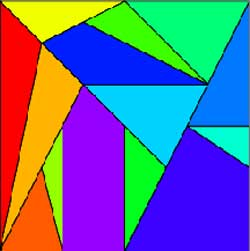People used computers to show that the total number is $17152=2^8 \times 67$. The 2-power is hardly surprising in view of symmetries of the square (giving $8$) and the fact that one can flip one of the two vertical or diagonal parts in the alternative description of the squarebut I sure would like to know where the factor 67 is coming from… The MAA and UCSD have some good pages related to the stomachion puzzle. Finally, the book also views the problema bovinum as an authentic Archimedes, so maybe I should stick to my promise to blog about it, after all…

Below an up-till-now hidden post, written november last year, trying to explain the long blog-silence at neverendingbooks during october-november 2007…

A couple of months ago a publisher approached me, out of the blue, to consider writing a book about mathematics for the general audience (in Dutch (?!)). Okay, I brought this on myself hinting at the possibility in this post

Recently, I’ve been playing with the idea of writing a book for the general public. Its title is still unclear to me (though an idea might be “The disposable science”, better suggestions are of course wellcome) but I’ve fixed the subtitle as “Mathematics’ puzzling fall from grace”. The book’s concept is simple : I would consider the mathematical puzzles creating an hype over the last three centuries : the 14-15 puzzle for the 19th century, Rubik’s cube for the 20th century and, of course, Sudoku for the present century.

For each puzzle, I would describe its origin, the mathematics involved and how it can be used to solve the puzzle and, finally, what the differing quality of these puzzles tells us about mathematics’ changing standing in society over the period. Needless to say, the subtitle already gives away my point of view. The final part of the book would then be more optimistic. What kind of puzzles should we promote for mathematical thinking to have a fighting chance to survive in the near future?

While I still like the idea and am considering the proposal, chances are low this book ever materializes : the blog-title says it all…

Then, about a month ago I got some incoming links from a variety of Flemish blogs. From their posts I learned that the leading Science-magazine for the low countries, Natuur, Wetenschap & Techniek (Nature, Science & Technology), featured an article on Flemish science-blogs and that this blog might be among the ones covered. It sure would explain the publisher’s sudden interest. Of course, by that time the relevant volume of NW&T was out of circulation so I had to order a backcopy to find out what was going on. Here’s the relevant section, written by their editor Erick Vermeulen (as well as an attempt to translate it)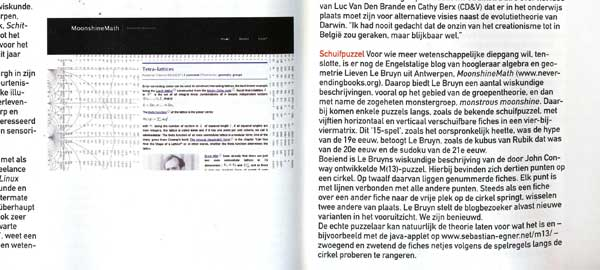Sliding puzzle For those who want more scientific depth (( their interpretation, not mine )), there is the English blog by Antwerp professor algebra & geometry Lieven Le Bruyn, MoonshineMath (( indicates when the article was written… )). Le Bruyn offers a number of mathematical descriptions, most of them relating to group theory and in particular the so called monster-group and monstrous moonshine. He mentions some puzzles in passing such as the well known sliding puzzle with 15 pieces sliding horizontally and vertically in a 4 by 4 matrix. Le Bruyn argues that this ’15-puzzle (( The 15-puzzle groupoid ))’ was the hype of the 19th century as was the Rubik cube for the 20th and is Sudoku for the 21st century.
Interesting is Le Bruyn’s mathematical description of the M(13)-puzzle (( Conway’s M(13)-puzzle )) developed by John Conway. It has 13 points on a circle, twelve of them carrying a numbered counter. Every point is connected via lines to all others (( a slight simplification )). Whenever a counter jumps to the empty spot, two others exchange places. Le Bruyn promises the blog-visitor new variants to come (( did I? )). We are curious.
Of course, the genuine puzzler can leave all this theory for what it is, use the Java-applet (( Egner’s M(13)-applet )) and painfully try to move the counters around the circle according to the rules of the game.

Some people crave for this kind of media-attention. On me it merely has a blocking-effect. Still, as the end of my first-semester courses comes within sight, I might try to shake it off…